### The Lorentz Force from the Classical Hamiltonian

In this section, we wish to verify that the Hamiltoniangives the correct Lorentz Force law in classical physics. We will then proceed to use this Hamiltonian in Quantum Mechanics.

Hamilton's equations arewhere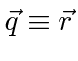and the conjugate momentum is already identified correctly. Remember that these are applied assuming q and p are independent variables.

Beginning with, we haveNote that. The momentum conjugate to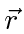includes momentum in the field. We now time differentiate this equation and write it in terms of the components of a vector.Similarly for the other Hamilton equation (in each vector component)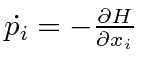, we have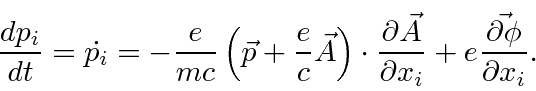We now have two equations for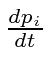derived from the two Hamilton equations. We equate the two right hand sides yieldingThe total time derivative of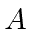has one part fromchanging with time and another from the particle moving andchanging in space.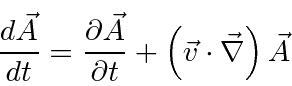so thatWe notice the electric field term in this equation.Let's work with the other two terms to see if they give us the rest of the Lorentz Force.We need only prove thatTo prove this, we will expand the expression using the totally antisymmetric tensor.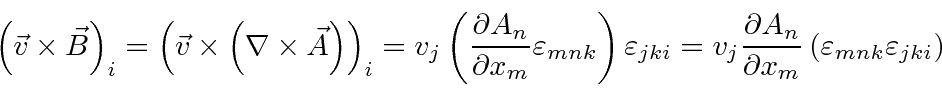Q.E.D.

So we havewhich is the Lorentz force law. So this is the right Hamiltonian for an electron in a electromagnetic field. We now need to quantize it.

Jim Branson 2013-04-22